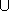## LinearProgram

### Definition

A model of LinearProgram describes a linear program of the form



 (QP) minimize cTx+c0 subject to Ax ~ b, lxu

in n real variables x=(x0,...,xn-1). Here,

• A is an m × n matrix (the constraint matrix),
• b is an m-dimensional vector (the right-hand side),
•  ~  is an m-dimensional vector of relations from {, =,},
• l is an n-dimensional vector of lower bounds for x, where lj{-} for all j
• u is an n-dimensional vector of upper bounds for x, where uj{} for all j
• c is an n-dimensional vector (the linear objective function), and
• c0 is a constant.

The description is given by appropriate random-access iterators over the program data, see below. The program therefore comes in dense representation which includes zero entries.

### Has Models

Linear_program_from_iterators<A_it, B_it, R_it, FL_it, L_it, FU_it, U_it, D_it, C_it>

### Types

 LinearProgram::A_iterator A random access iterator type to go columnwise over the constraint matrix A. The value type is a random access iterator type for an individual column that goes over the entries in that column. LinearProgram::B_iterator A random access iterator type to go over the entries of the right-hand side b. LinearProgram::R_iterator A random access iterator type to go over the relations  ~ . The value type of R_iterator is CGAL::Comparison_result. LinearProgram::FL_iterator A random access iterator type to go over the existence (finiteness) of the lower bounds lj, j=0,...,n-1. The value type of FL_iterator is bool. LinearProgram::L_iterator A random acess iterator type to go over the entries of the lower bound vector l. LinearProgram::UL_iterator A random access iterator type to go over the existence (finiteness) of the upper bounds uj, j=0,...,n-1. The value type of UL_iterator is bool. LinearProgram::U_iterator A random acess iterator type to go over the entries of the upper bound vector u. LinearProgram::C_iterator A random access iterator type to go over the entries of the linear objective function vector c.

### Operations

int lp.get_n () returns the number n of variables (number of columns of A) in lp.

int lp.get_m () returns the number m of constraints (number of rows of A) in lp.

A_iterator lp.get_a () returns an iterator over the columns of A. The corresponding past-the-end iterator is get_a()+get_n(). For j=0,...,n-1, *(get_a()+j) is a random access iterator for column j.

B_iterator lp.get_b () returns an iterator over the entries of b. The corresponding past-the-end iterator is get_b()+get_m().

R_iterator lp.get_r () returns an iterator over the entries of  ~ . The corresponding past-the-end iterator is get_r()+get_m(). The value CGAL::SMALLER stands for, CGAL::EQUAL stands for =, and CGAL::LARGER stands for.

FL_iterator lp.get_fl () returns an iterator over the existence of the lower bounds lj, j=0,...,n-1. The corresponding past-the-end iterator is get_fl()+get_n(). If *(get_fl()+j) has value true, the variable xj has a lower bound given by *(get_l()+j), otherwise it has no lower bound.

L_iterator lp.get_l () returns an iterator over the entries of l. The corresponding past-the-end iterator is get_l()+get_n(). If *(get_fl()+j) has value false, the value *(get_l()+j) is not accessed.
 Precondition: if both *(get_fl()+j) and *(get_fu()+j) have value true, then *(get_l()+j)*(get_u()+j)

FU_iterator lp.get_fu () returns an iterator over the existence of the upper bounds uj, j=0,...,n-1. The corresponding past-the-end iterator is get_fu()+get_n(). If *(get_fu()+j) has value true, the variable xj has an upper bound given by *(get_u()+j), otherwise it has no upper bound.

L_iterator lp.get_u () returns an iterator over the entries of u. The corresponding past-the-end iterator is get_u()+get_n(). If *(get_fu()+j) has value false, the value *(get_u()+j) is not accessed.
 Precondition: if both *(get_fl()+j) and *(get_fu()+j) have value true, then *(get_l()+j)*(get_u()+j)

C_iterator lp.get_c () returns an iterator over the entries of c. The corresponding past-the-end iterator is get_c()+get_n().

std::iterator_traits<C_iterator>::value_type
lp.get_c0 () returns the constant term c0 of the objective function.